## Friday, April 29, 2011

### 340

340 = 22 x 5 x 17.

340 has two representations as a sum of two squares: 340 = 42 + 182 = 122 + 142.

340 is the number of integer partitions of 31 into distinct parts.

340 is a divisor of 692 - 1.

340 is a value of n for which n! + 1 is a prime.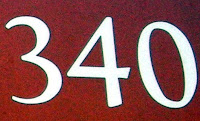The Airbus A340 is a long-range, four-engine wide-body commercial passenger jet airliner.

## Thursday, April 28, 2011

### 130

130 = 2 x 5 x 13.

130 has two representations as a sum of two squares: 130 = 32 + 112 = 72 + 92.

130 is the smallest number with eight representations as a sum of four positive squares: 130 = 12 + 12 + 82 + 82 = 12 + 22 + 22 + 112 = 12 + 22 + 52 + 102 = 12 + 42 + 72 + 82 = 22 + 32 + 62 + 92 = 32 + 62 + 62 + 72 = 42 + 42 + 72 + 72 = 42 + 52 + 52 + 82.

130 is a cake number.

130 is the sum of the factorials of the first five terms of the Fibonacci sequence: 130 = 1! + 1! + 2! + 3! + 5!.

130 is the only number that is equal to the sum of the squares of its first four divisors: 130 = 12 + 22 + 52 + 102.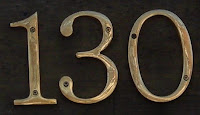The Lockheed C-130 Hercules is a four-engine turboprop military transport aircraft.

## Wednesday, April 27, 2011

### 126

126 = 2 x 32 x 7.

126 is the sixth pentatope number.

126 is 99 in base 13.

126 is the number of prime days in a non-leap year.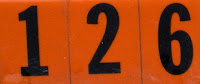The Ferrari 126C was Ferrari's first attempt at turbo-charged Formula 1 car.

## Tuesday, April 26, 2011

### 263

263 is a prime number.

263 is the largest known prime whose square is strobogrammatic; that is, it is the same number when rotated 180 degrees: 2632 = 69169.

263 is the smallest prime number that is the sum of primes beginning and ending with the digit 1: 263 = 11 + 101 + 151. Note that the digit sum of each prime is prime and that the digit sum of 263 is a prime and begins and ends with the digit 1.

263 is the only prime with three distinct digits, where one is the product of the other two.

263 is the smallest prime formed be inserting a semiprime, 6, between the semiprime's factors, 2 and 3.

263 is a number that cannot be written as a sum of three squares.Anne Frank (and her family) hid from the Nazis in an annex behind the house at 263 Prinsengracht 263 in Amsterdam.

## Monday, April 25, 2011

### 855

855 = 32 x 56 x 19.

855 is the sum of all the digits of all two-digit numbers.

855 is a number that cannot be written as a sum of three squares.

855 is a decagonal number. It is also a centered cube number.

855 is the smallest number that is the sum of five consecutive squares and two consecutive cubes: 855 = 112 + 122 + 132 + 142 + 152 = 73 + 83.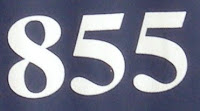855 Newcombia is a asteroid discovered in 1916.

## Friday, April 22, 2011

### 430

430 = 2 x 5 x 43.

430 is the number of necklaces possible with six beads, each being one of four colors.

430 is 656 in base 8.

430 is the number of primes less than 3000.

430 is a number such that its square is the sum of two or more consecutive squares.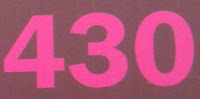The Ferrari F430 is a sports car produced from 2004 to 2009.

## Thursday, April 21, 2011

### 6011

6011 is a prime number. Rotating 6011 by 180 degrees (turning it upside down) produces 1109, another prime.

6011 is a member of the Fibonacci-like sequence starting with 3 and 8.

6011 is a prime p such that the product its digits (excluding 0) is a divisor of p + 1.

60112 uses only the digits 1, 2, 3, and 6: 36132121.

60/11 equals 5.4545. . . , the exact time difference between consecutive overlaps of the hour and minute hands, which occurs 11 times every 60 minutes.6011 is the postal code for Peppermint Grove, Western Australia, a suburb of Perth.

## Wednesday, April 20, 2011

### 600

600 = 23 x 3 x 52.

600 is the product of two consecutive integers, so it is a pronic number: 600 = 24 x 25.

600 and its reverse, 6, are both the averages of twin primes: 599 and 601; 5 and 7.

600 is 211020 in base 3 and 21120 in base 4; it is 4400 in base 5, 2440 in base 6, and 420 in base 12; it is 1515 in base 7.600 is the value of the postal abbreviation for the District of Columbia, DC, in the United States, interpreted as Roman numerals.

## Tuesday, April 19, 2011

### 298

298 = 2 x 149.

298 has a representation as a sum of two squares: 298 = 32 + 172.

298 = (22 + 92 + 82) + (22 + 92 + 82).

298 is the sum of four distinct squares a2 + b2 + c2 + d2, such that a2 + b2, c2 + d2, a2 + d2, and b2 + c2 are all prime.298 Baptistina is an asteroid discovered in 1890.

Source: Numeropedia

## Monday, April 18, 2011

### 335

335 = 5 x 67. It is divisible by the number of primes below it (67).

335 is a number that cannot be written as a sum of three squares.

335 is a divisor of 662 - 1.

2335 is the smallest power of 2 that equals the sum of four consecutive primes.

335 is 656 in base 7.The ES-335, a semi-acoustic electric guitar, was produced in 1958 by the Gibson Guitar Corp.

## Friday, April 15, 2011

### 457

457 is a prime number.

457 has a representation as a sum of two squares: 457 = 42 + 212.

457 is the hypotenuse of a primitive Pythagorean triple: 4572 = 1682 + 4252.

457 is a divisor of 1812 - 1.

457 is the index of a prime Euclid number.

457 is the sum of three consecutive primes: 457 = 149 + 151 + 157.The German submarine known as U-457 was sunk in the Barents Sea by depth charges from the British destroyer HMS Impulsive during World War II.

## Thursday, April 14, 2011

### 599

599 is a prime number.

599 and 601 form a twin prime pair.

599 is a number that cannot be written as a sum of three squares.

599 is a divisor of 1913 - 1.

599 is the smallest number whose digits add to 23.The Administrative building in the town of Saint-Prime, Quebec, is at the street address 599, rue Principale.

## Wednesday, April 13, 2011

### 445

445 = 5 x 89.

445 has two representations as a sum of two squares: 445 = 22 + 212 = 112 + 182.

445 is the hypotenuse of two primitive Pythagorean triples: 4452 = 842 + 4372 = 2032 + 3962.

445 is a divisor of 344 - 1.

445 has a base 10 representation (445) that is the reverse of its base 9 representation (544).The Skoda 445 automobile was produced in Czechoslovakia between 1955 and 1959.

## Tuesday, April 12, 2011

### 1237

1237 is a prime number.

1237 has a representation as a sum of two squares: 1237 = 92 + 372.

1237 is the hypotenuse of a primitive Pythagorean triple: 12372 = 6122 + 12752.

12372 - (1237 - 5)2 = 12345.

1237 is also a prime number when its digits are reversed (emirp): 7321. It is the smallest 4-digit emirp with distinct digits. The sum of its digits, 1 + 2 + 3 + 7 = 13, is equal to the smallest double-digit emirp. 1237 is an emirp formed from the number 12 and the 12th prime; its reversal (7321) is formed from the 21st prime and the number 21.1237 Geneviève is an asteroid discovered in 1931.

## Monday, April 11, 2011

### 179

179 is a prime number. 179 is a Sophie Germain prime because 2 x 179 + 1 = 359, a prime.

179 and 181 form a twin prime pair.

179 = (17 x 9) + (17 + 9).

179 has a square comprised of the digits 0 to 4: 1792 = 32041.

179 x 79 = 14141.There are 179 even days in one year.

## Friday, April 8, 2011

### 686

686 = 2 x 73.

686 is a divisor of 196 - 1.

686 is the number of partitions of 35 in which no part occurs only once.

686 is 2000 in base 7 and 222 in base 18.686 Clothing produces fashionable sportswear.

## Thursday, April 7, 2011

### 861

861 = 3 x 7 x 41.

861 is the 41st triangular number. It is also a hexagonal number.

861 = 7 + 77 + 777.

144,648 = 861 x 168 = 492 x 294.The USS Harwood (DD 861) was a destroyer in the U.S. Navy just after World War II.

## Wednesday, April 6, 2011

### 477

477 = 32 x 53,

477 has a representation as a sum of two squares: 477 = 62 + 212.

477 is the smallest number that is the sum of different primes by using twice all the digits greater than 0: 477 = 89 + 83 + 67 + 61 + 53 + 47 + 41 + 29 + 5 + 2.

477 is the smallest number whose cube contains four 3s: 4773 = 108,531,333.Masonic Funeral Music in C by Wolfgang Amadeus Mozart is cataloged as KV 477.

## Tuesday, April 5, 2011

### 380

380 = 22 x 5 x 19.

380 is a pronic number because it is the product of two consecutive numbers: 380 = 19 x 20. Hence, it is also twice a triangular number.

380 is the smallest number divisible by 5 of the form p(p + 1) where p is a prime and doesn't equal 5.

380 is a number that cannot be written as a sum of three squares.

380 is the number of necklaces possible with 13 beads, each being one of two possible colors.The Airbus A380 aircraft, the largest commercial aircraft flying today, was introduced in the year 2007.

## Monday, April 4, 2011

### 62

62 = 2 x 31.

62 is 222 in base 5.

62 = 14 + 15 + 16 + 17.

62 is the smallest number that can be written as the sum of three distinct squares in two ways: 62 = 72 + 32 + 22 = 62 + 52 + 12.

62 is the only number whose cube (238328) consists of three digits, each occurring two times.A truncated icosidodecahedron has 62 faces (12 decagons, 20 hexagons, and 30 squares).

Source: Wikipedia

## Friday, April 1, 2011

### 668

668 = 22 x 167.

668 is the number of integer partitions of 36 into distinct parts.

668 is a number that cannot be written as a sum of three squares.

668 = 36 - 61.

334,668 = 6682 - 3342 = (334 + 668) x 334, where 668 = 2 x 334.668 is the number of legal pawn moves in chess.